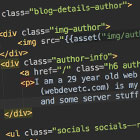## PHP: How to check if two strings are anagrams in PHP?

This is a classic simple programming question. There are several ways to approach this problem. Here is one solution:

The comparison function:

`function is_anagram(\$a, \$b){     if (strlen(\$a) != strlen(\$b)) {        // if not same size then they definitely aren't        return false;    } // turn 'foobar' into ['f', 'o', 'o', 'b', 'a', 'r']\$a_chars = str_split(\$a);\$b_chars = str_split(\$b); // sort them...sort(\$a_chars);sort(\$b_chars); // check if they're exactly the same...return \$a_chars === \$b_chars; // another way to do this that someone emailed me about, which is much more simple:// return (count_chars(\$a, 1) == count_chars(\$b, 1))  }`

Let's write some tests

`\$anagrams = [     // \$a, \$b, \$is_valid_anagram         ["asdf", "asdf", true],        ["asdf", "fdsa", true],        ["asdfasdf", "asdfasdf", true],        ["a", "a", true],        ["a", "b", false],        ["aaa", "aaa", true],        ["aaa", "aa", false],        ["aaaa", "abab", false],        ["qwerty", "asdfg", false] ];`

And now let's go through each one.

`foreach (\$anagrams as \$anagram) {     [\$a, \$b, \$is_valid_anagram] = \$anagram;     \$result = is_anagram(\$a, \$b);    \$result_message = \$result ? "Is an anagram" : "Is not an anagram";     if (\$result != \$is_valid_anagram) {        throw new \Exception("The anagram function returned an incorrect result for \$a and \$b");  // this doesn't happen with our function! :)    }    var_dump("[\$a] and [\$b] \$result_message"); }`

```"Our function said that [asdf] and [asdf] Is an anagram, which is correct"
"Our function said that [asdf] and [fdsa] Is an anagram, which is correct"
"Our function said that [asdfasdf] and [asdfasdf] Is an anagram, which is correct"
"Our function said that [a] and [a] Is an anagram, which is correct"
"Our function said that [a] and [b] Is not an anagram, which is correct"
"Our function said that [aaa] and [aaa] Is an anagram, which is correct"
"Our function said that [aaa] and [aa] Is not an anagram, which is correct"
"Our function said that [aaaa] and [abab] Is not an anagram, which is correct"
"Our function said that [qwerty] and [asdfg] Is not an anagram, which is correct"```

BTW, not sure about how the ` [\$a, \$b, \$is_valid_anagram] = \$anagram;` line works? See my blog post about array destructuring in PHP 7.webdevetc

I am a 29 year old backend web developer from London, mostly focusing on PHP and Laravel lately. This (webdevetc.com) is my blog where I write about some web development topics (PHP, Laravel, Javascript, and some server stuff). contact me here.

### More...

##### Or see other languages/frameworks:
PHP Laravel Composer Apache CentOS and Linux Stuff WordPress General Webdev and Programming Stuff JavaScript